• 在简单的VC6.0下开发的C语言程序，是对于数据结构集合运算的简单实现
• 调试要求：1 实验目的本实验是要实现求集合（用单链表表示）的并、交和差运算，通过该实验更深刻地理解线性结构的链表的特点。 2 实验内容实现集合（用单链表表示）的并、交和差运算，并在此基础上设计一个主程序...
实验思路1.构建链表；2.各个功能的函数3.调试要求：1 实验目的本实验是要实现求集合（用单链表表示）的并、交和差运算，通过该实验更深刻地理解线性结构的链表的特点。 2 实验内容实现集合（用单链表表示）的并、交和差运算，并在此基础上设计一个主程序完成以下功能：(1) 初始化集合A{'c','a','e','h'}、B{'f','h','b','g','d','a'}和空集合C(2) 分别创建三个链表分别存放集合A、B和C(3) 输出集合A和B的并运算结果(4) 输出集合A和B的交运算结果(5) 输出集合A和B的差运算结果(6) 释放三个链表具体代码如下：#include<stdio.h>
#include<stdlib.h>

//******宏定义参数******
#define OK 1
#define NO 0
#define DATA_MAX_SIZE 20

//******定义数据类型别名******
typedef int Status;
typedef char Excelelem;
typedef int Numelem;

//******声明数据结构******
typedef struct Node
{
Excelelem book;
struct Node *next;
}LNode,*LinkList;

typedef struct
{
Excelelem name;
Numelem length;
LinkList next;
}HeadList,*HeadLinkList;

//******初始化链表******
LinkList init(int *i)
{
LinkList Head,p,q;
Excelelem ch;
Head=q=NULL;
while((ch=getchar())!='\n')
{
p=(LinkList)malloc(sizeof(LNode));
p->book=ch;
if (!(*i)) Head=q=p,(*i)++;
else
{
q->next=p;
q=p;
(*i)++;
}
}
if(p) p->next=NULL;
return Head;
}

HeadLinkList HeadInit()
{
//省略表头信息  Head->name
HeadLinkList Head;
Head=(HeadLinkList)malloc(sizeof(HeadList));
Head->length=0;
Head->next=init(&Head->length);
return Head;
}

//******输出链表中的信息******
void DATA_cout(HeadLinkList Head)
{
LinkList p=Head->next;
while(p!=NULL)
{
printf("%c",p->book);
p=p->next;
}
printf("\n");
return ;
}

//******返还内存******
void DATA_Free(HeadLinkList Head)
{
LinkList q=Head->next,p;
while (q!=NULL)
{
p=q;
q=q->next;
free(p);
}
Head->length=0;
Head->next=NULL;
return ;
}

//******在i位置之前插入一个元素ch******
void DATA_Insert(HeadLinkList Head,Numelem k,Excelelem ch)
{
int i=1;
LinkList q=Head->next,p,t;
if(Head->length && (Head->length<k || k<1))
{
printf("警告！%d位置不合法\n",k);
return ;
}
while(p && i++ < k)
p=q,q=q->next;
t=(LinkList)malloc(sizeof(LNode));
t->book=ch;
if(k==1)
{
Head->next=t;
t->next=q;
}
else
{
t->next=p;
q->next=t;
}
Head->length++;
return ;
}

//******查找是否出现字符ch******
int DATA_find(HeadLinkList Head,Excelelem ch)
{
LinkList q=Head->next;
while(q!=NULL && q->book!=ch)
q=q->next;
if(q==NULL)
return NO;
return OK;
}

//******AB******
void SetJiao(HeadLinkList A,HeadLinkList B,HeadLinkList C)
{
LinkList q=A->next;
DATA_Free(C); //初始化结果集合C，防止内存泄漏
while (q!=NULL)
{
if(DATA_find(B,q->book) && !DATA_find(C,q->book))
DATA_Insert(C,1,q->book);
q=q->next;
}
return ;
}

//******A+B******
void SetBing(HeadLinkList A,HeadLinkList B,HeadLinkList C)
{
LinkList q=A->next;
DATA_Free(C);
while(q!=NULL)
{
if(!DATA_find(C,q->book))
DATA_Insert(C,1,q->book);
q=q->next;
}
q=B->next;
while(q!=NULL)
{
if(!DATA_find(C,q->book))
DATA_Insert(C,1,q->book);
q=q->next;
}
return ;
}

//******A-B******
void SetCha(HeadLinkList A,HeadLinkList B,HeadLinkList C)
{
LinkList q=A->next;
DATA_Free(C);
while(q!=NULL)
{
if(!DATA_find(B,q->book) && !DATA_find(C,q->book))
DATA_Insert(C,1,q->book);
q=q->next;
}
return ;
}

/***************************
以上为O(n*n)算法
还有一种为O(n)算法,即在构建集合时预处理，把集合的信息映射到一张ASCLL表中，之后直接查表，免去了遍历链表的步骤,为了之后
的查表不做多余判断，在预处理的时候，要记录数据的边界

交运算:
if book[ch]==2
C.Insert(ch);
并运算:
if book[ch]!=0
C.Insert(ch);
差运算:
if book[ch]==1
C.Insert(ch);

*****************************/

int main()
{
HeadLinkList A,B,C;
C=(HeadLinkList)malloc(sizeof(HeadList));
C->next=NULL;
C->length=0;
printf("******初始化集合A******\n");
A=HeadInit();
printf("******初始化集合B******\n");
B=HeadInit();
printf("*****集合AB的结果*****\n");
SetJiao(A,B,C);
DATA_cout(C);
printf("*****集合A+B的结果*****\n");
SetBing(A,B,C);
DATA_cout(C);
printf("*****集合A-B的结果*****\n");
SetCha(A,B,C);
DATA_cout(C);
printf("***********************\n");
printf("*******释放内存*******\n");
DATA_Free(A),DATA_Free(B),DATA_Free(C);
free(A),free(B),free(C);
printf("**********End!**********\n");
return 0;
}
总结：实现集合运算有挺多方法的，其具体步骤包括查找和去重，严蔚敏《数据结构C语言版》书上还有一种算法，不过也是O(n*n)，思路和上面的代码基本相似，但如果输入的字符ASCLL差别不大，可以建表，这样就能一劳永逸，不过如果输入的数据较为稀疏这样时间就没啥区别了，甚至O(n*n)的还会快点，具体看要求而定！
展开全文• 本课程设计中，集合的元素限定为小写字母...2. 链表L包括数据域和指针域，数据域中存储集合元素，指针域中存储下一个集合元素的位置； 3. 创建若干个基本函数，通过函数调用对链表进行作，实现集合的交、并、差运算
• 数据结构集合运算，运用C语言描述的数据结构来实现集合的基本运算包括并交差。
• 在C++6.0环境下编写的关于数据结构的课程设计，附带课程设计报告C++
• 数据结构课程设计—集合运算。用于数据结构的课程设计。欢迎下载。
• 集合运算 功能：使用链表来表示集合，完成集合的交、并、差等操作。 主要包含以下内容： 1、初步完成总体设计，搭好框架，确定人机对话的界面，确定函数个数； 2、完成最低要求：完成集合的各种基本运算； 代码 ...
集合运算
功能：使用链表来表示集合，完成集合的交、并、差等操作。
主要包含以下内容：
1、初步完成总体设计，搭好框架，确定人机对话的界面，确定函数个数；
2、完成最低要求：完成集合的各种基本运算；
代码
linklist.cpp
#include<stdio.h>
#include<malloc.h>

typedef char ElemType;

typedef struct LNode
{
ElemType data;                                    //存放元素值
struct LNode *next;                               //指向后继结点
}LinkNode;		                                      //声明单链表节点类型

void CreateListF(LinkNode *&L,ElemType a[],int n)     //头插法建立单链表
{
LinkNode *s;
L=(LinkNode *)malloc(sizeof(LinkNode));           //创建头结点
L->next=NULL;                                     //创建头结点，其next域值为NULL
for(int i=0;i<n;i++)                              //循环建立数据结点s
{
s=(LinkNode *)malloc(sizeof(LinkNode));       //创建新结点
s->data=a[i];                              	  //创建数据结点s
s->next=L->next;                              //将结点s插在原开始结点之前，头结点之后
L->next=s;
}
}

void CreateListR(LinkNode *&L,ElemType a[],int n)     //尾插法建立单链表
{
LinkNode *s,*r;
L=(LinkNode *)malloc(sizeof(LinkNode));           //创建头结点
L->next=NULL;
r=L;			                                  //r始终指向尾结点，开始是指向头结点
for(int i=0;i<n;i++)                              //循环建立数据结点s
{
s=(LinkNode *)malloc(sizeof(LinkNode));       //创建新结点s
s->data=a[i];                                 //创建数据结点s
r->next=s;                                    //将结点s插入r结点之后
r=s;
}
r->next=NULL;                                     //尾结点的next域值为NULL
}

void InitList(LinkNode *&L)                           //初始化线性表
{
L=(LinkNode*)malloc(sizeof(LinkNode));            //创建头结点
L->next=NULL;                                     //创建头结点，其next域值为NULL
}

void DestroyList(LinkNode *&L)                        //销毁线性表
{
LinkNode *pre=L, *p=pre->next;                    //pre指向结点p的前驱结点
while(p!=NULL)                                    //扫描单链表L
{
free(pre);                                    //释放pre结点
pre=p;                                        //pre和p同步后移一个结点
p=pre->next;
}
free(pre);                                        //循环结束时p为NULL,pre指向尾结点，释放它
}

bool ListEmpty(LinkNode *L)                           //判断线性表是否为空
{
return(L->next==NULL);
}

int ListLength(LinkNode *L)                           //求线性表的长度
{
int i=0;
LinkNode *p=L;                                    //p指向头结点，n置为0（即头结点的序号为0）
while(p->next!=NULL)
{
i++;
p=p->next;
}
return i;                                         //循环结束时，p指向尾结点，其序号n为结点个数
}

void DispList(LinkNode *L)                            //输出线性表
{
LinkNode *p=L->next;                              //p指向首结点
while(p!=NULL)                                    //p不为NULL，输出p结点的data域
{
printf("%c ", p->data);
p=p->next;                                    //p移向下一个结点
}
printf("\n");
}

bool GetElem(LinkNode *L,int i,ElemType &e)           //求线性表中的某个数据元素值
{
int j=0;
LinkNode *p=L;                                    //p指向头结点，j置为0（即头结点的序号）
if(i<=0)
return false;                                 //i错误，返回false
while(j<i&&p!=NULL)                               //找第i个结点
{
j++;
p=p->next;
}
if(p==NULL)                                       //不存在第i个结点
return false;
else                                              //存在第i个结点
{
e=p->data;                                    //当j=i时，将这个元素的值赋给e
return true;
}
}

int LocateElem(LinkNode *L,ElemType e)                //按元素查找
{
int i=1;
LinkNode *p=L->next;                              //p指向首结点，i置1（即首结点的序号为1）
while(p!=NULL&&p->data!=e)                        //查找data值为1的结点，其序号为i
{
p=p->next;
i++;
}
if(p==NULL)                                       //如果不存在值为e的结点，则返回0
return 0;
else                                              //如果存在值为e的结点，则返回逻辑序号i
return i;
}

bool ListInsert(LinkNode *&L,int i,ElemType e)        //插入第i个数据元素
{
int j=0;
LinkNode *p=L,*s;                                 //指向头结点，j置0（即头结点的序号为0）
if(i<=0)
return false;
while (j<i-1&&p!=NULL)                            //查找第i-1个结点
{
j++;
p=p->next;
}
if(p==NULL)                                       //如果未找到第i-1个结点，则返回false
return false;
else                                              //如果找到第i-1个结点p，则插入新结点并返回true
{
s=(LinkNode*)malloc(sizeof(LinkNode));
s->data=e;                                    //创建新结点s,其域值为e
s->next=p->next;                              //将s结点插入到结点p之后
p->next=s;
return true;
}
}

bool ListDelete(LinkNode *&L,int i,ElemType &e)       //删除第i个元素
{
int j=0;
LinkNode *p=L,*q;                                 //p指向头结点，j置为0（即头结点的序号为0）
if(i<=0)
return false;                                 //i错误，返回false
while(j<i-1&&p!=NULL)                             //查找第i-1个结点
{
j++;
p=p->next;
}
if (p==NULL)                                      //如果未找到第i-1个结点，则返回false
return false;
else                                              //找到第i - 1个结点p
{
q=p->next;                                    //q指向第i个结点
if(q==NULL)                                   //如果不存在第i个结点，则返回false
return false;
e=q->data;
p->next=q->next;                              //从单链表中删除q结点
free(q);                                      //释放q结点
return true;                                  //成功删除第i个结点，则返回true
}
}

operation.cpp
#include "linklist.cpp"
#include "string.h"
#include "stdlib.h"
#define MaxSize 20                                    //可根据需要改变MaxSize的数值
void Sort(LinkNode *&L)                                    //单链表元素递增排序
{
LinkNode *p, *pre, *q;
p = L->next->next;                                     //p指向L的第2个数据节点
L->next->next = NULL;                                  //构造只含一个数据节点的有序表
while (p != NULL)
{
q = p->next;                                       //q保存p节点的后继节点
pre = L;                                           //从有序表开头进行比较，pre指向插入节点p的前驱节点
while (pre->next != NULL && pre->next->data < p->data)
pre = pre->next;                               //在有序表中找pre节点
p->next = pre->next;                               //在节点pre之后插入p节点
pre->next = p;
p = q;                                             //扫描原单链表余下的节点
}
}
void Union(LinkNode *ha, LinkNode *hb, LinkNode *&hc)      //求两个有序集合的并
{
LinkNode *pa = ha->next, *pb = hb->next, *s, *tc;
hc = (LinkNode *)malloc(sizeof(LinkNode));             //创建头结点
tc = hc;
while (pa != NULL && pb != NULL)
{
if (pa->data < pb->data)
{
s = (LinkNode *)malloc(sizeof(LinkNode));      //复制节点
s->data = pa->data;
tc->next = s;
tc = s;
pa = pa->next;
}
else if (pa->data > pb->data)
{
s = (LinkNode *)malloc(sizeof(LinkNode));      //复制节点
s->data = pb->data;
tc->next = s;
tc = s;
pb = pb->next;
}
else
{
s = (LinkNode *)malloc(sizeof(LinkNode));      //复制节点
s->data = pa->data;
tc->next = s;
tc = s;
pa = pa->next;                                 //重复的元素只复制一个节点
pb = pb->next;
}
}
if (pb != NULL)
pa = pb;                                           //复制余下的节点
while (pa != NULL)
{
s = (LinkNode *)malloc(sizeof(LinkNode));          //复制节点
s->data = pa->data;
tc->next = s;
tc = s;
pa = pa->next;
}
tc->next = NULL;
}
void InterSect(LinkNode *ha, LinkNode *hb, LinkNode *&hc)  //求两个有序集合的交
{
LinkNode *pa = ha->next, *pb, *s, *tc;
hc = (LinkNode *)malloc(sizeof(LinkNode));
tc = hc;
while (pa != NULL)
{
pb = hb->next;
while (pb != NULL && pb->data < pa->data)//已经是有序链表，若pa中数据大于pb，pb往下移一个，寻找与pa数据相等节点
pb = pb->next;
if (pb != NULL && pb->data == pa->data)            //若pa节点值在B中
{
s = (LinkNode *)malloc(sizeof(LinkNode));      //复制节点
s->data = pa->data;
tc->next = s;
tc = s;
}
pa = pa->next;
}
tc->next = NULL;
}
void Subs(LinkNode *ha, LinkNode *hb, LinkNode *&hc)       //求两个有序集合的差
{
LinkNode *pa = ha->next, *pb, *s, *tc;
hc = (LinkNode *)malloc(sizeof(LinkNode));
tc = hc;
while (pa != NULL)
{
pb = hb->next;
while (pb != NULL && pb->data < pa->data)
pb = pb->next;
if (!(pb != NULL && pb->data == pa->data))         //若pa节点值不在B中
{
s = (LinkNode*)malloc(sizeof(LinkNode));       //复制节点
s->data = pa->data;
tc->next = s;
tc = s;
}
pa = pa->next;
}
tc->next = NULL;
}
int main()
{
LinkNode *ha, *hb, *hc;
ElemType a[MaxSize];
ElemType b[MaxSize];
int selection;
system("TITLE 集合运算操作系统");
system("color 04");
do  //让用户选择是否继续进行集合操作
{
printf("                       ┏━┓　┏━┓ \n");
printf("                      ★│┃  ┃│┃ \n");
printf("                       ┃│┗灬┛│┃___ \n");
printf("                       ┃　　　　　┃\n");
printf("                       ┃ ^　　 ^  ┃\n");
printf("                          ﹌ ˇ ﹌  \n");
printf("                       ┗○━━━○┛ \n");
printf("-------------------欢迎进入集合运算操作程序--------------------\n");
printf(">>请输入集合A:");scanf("%s", a);    //手动输入集合A
printf(">>请输入集合B:");scanf("%s", b);    //手动输入集合B
CreateListR(ha, a, strlen(a));  //尾插法建立单链表
CreateListR(hb, b, strlen(b));  //尾插法建立单链表
Sort(ha);Sort(hb);  //让单链表ha和hb中的元素递增排序
printf("\n            集合的运算如下:\n");
printf("****************************************\n");
printf("  集合的并为: ");Union(ha, hb, hc);DispList(hc);    //显示输出集合的并
printf("  集合的交为: ");InterSect(ha, hb, hc);DispList(hc);    //显示输出集合的交
printf("  集合的差为: ");Subs(ha, hb, hc);DispList(hc);//显示输出集合的差
printf("****************************************\n");
DestroyList(ha);DestroyList(hb);DestroyList(hc);    //销毁线性表ha、hb和hc
printf("提示：是否继续进行集合的运算:   *继续请按1  *退出请按任意键(除1外)\n");
printf(">>请输入你的选择：");
scanf("%d",&selection); //输入用户选择的操作
system("cls");  //清屏
}while(selection==1);//当输入1时继续集合操作，
return 1;
}


- 界面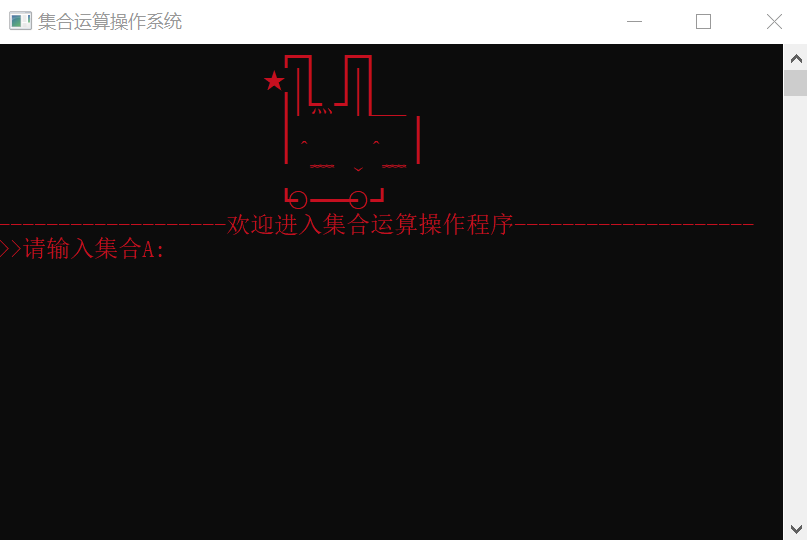- 测试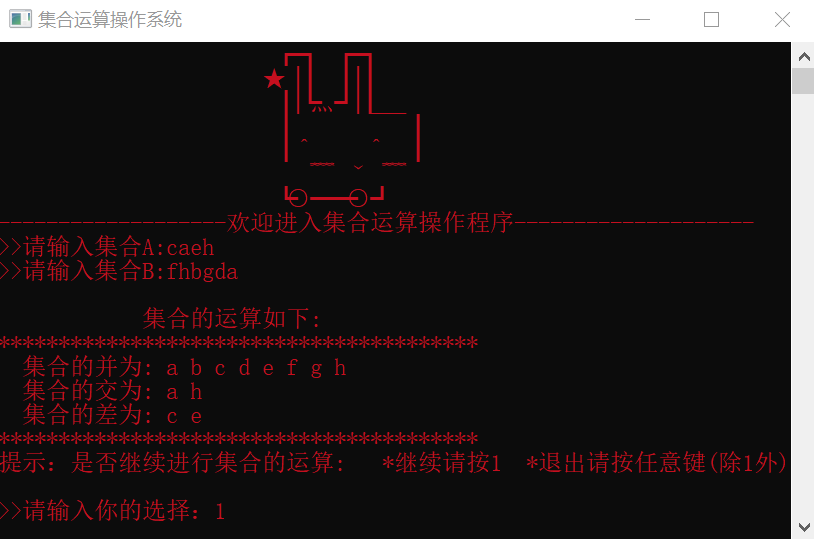P.S.这个代码还是存在缺陷的，用户输入的集合必须要连着输入，不可以有空格和逗号作为分隔符，否则会把空格和逗号也当成是一个字符进行运算，而且如果输入的是数字的话，这样就只可以进行单个数字的运算，无法完成多位数字的运算。我尝试过其他办法去修改这个缺陷，但是都失败了T_T终究还是太菜


展开全文• 转载于:https://www.cnblogs.com/geekqi/p/6083428.html转载于:https://www.cnblogs.com/geekqi/p/6083428.html
展开全文• 完整的数据结构课程设计书，主题是集合运算，包含了课程设计所需要的一切，目的要求啦，源代码啦，运行结果分析啦都有，让你的课程设计课轻松过，还不快下载，还在犹豫什么！
• 集合运算：交、并、补、差，判定一个元素是否属于某一集合 并查集：集合并、查某元素属于什么集合 并查集问题中集合存储如何实现？ 可以用树结构表示集合，树的每个结点代表一个集合元素 采用数组存储形式 ...
集合的表示
集合运算：交、并、补、差，判定一个元素是否属于某一集合
并查集：集合并、查某元素属于什么集合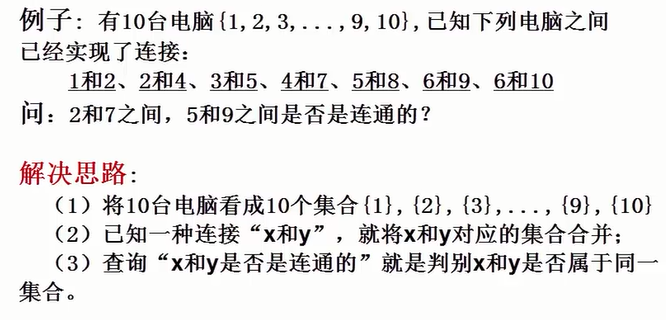并查集问题中集合存储如何实现？

可以用树结构表示集合，树的每个结点代表一个集合元素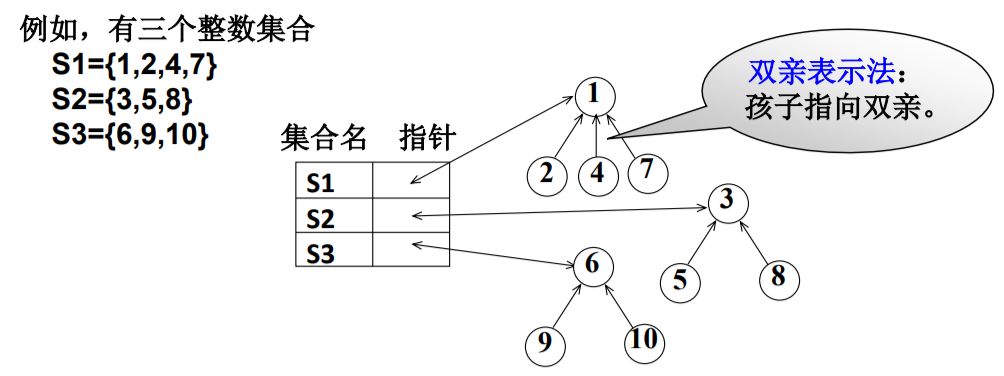采用数组存储形式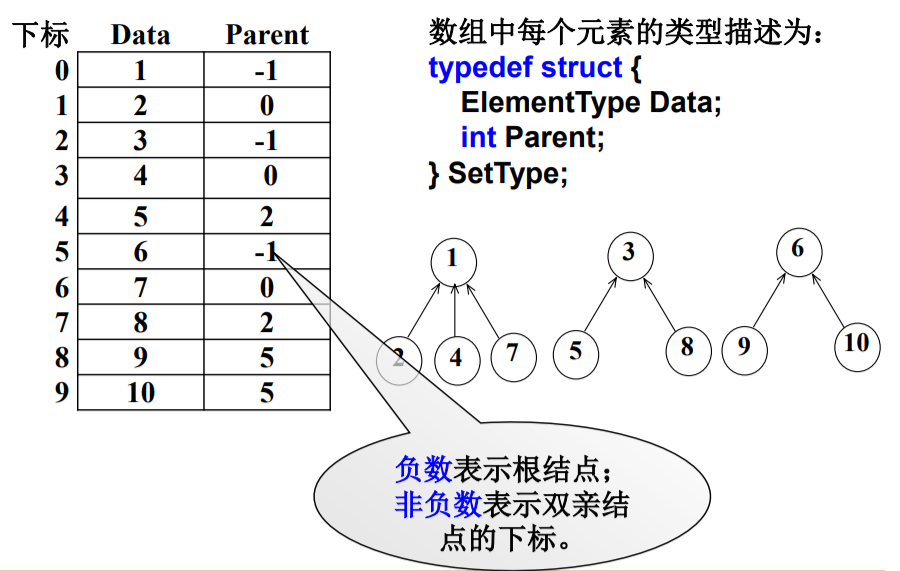集合运算
（1）查找某个元素所在的集合（用根结点表示）
int Find( SetType S[ ], ElementType X )
{ /* 在数组S中查找值为X的元素所属的集合 */
/* MaxSize是全局变量，为数组S的最大长度 */
int i;
for ( i=0; i < MaxSize && S[i].Data != X; i++) ;
if( i >= MaxSize ) return -1; /* 未找到X，返回-1 */
for( ; S[i].Parent >= 0; i = S[i].Parent ) ;
return i; /* 找到X所属集合，返回树根结点在数组S中的下标 */
}

（2）集合的并运算
分别找到X1和X2两个元素所在集合树的根结点
如果它们不同根，则将其中一个根结点的父结点指针设置成另一个根结点的数组下标。
void Union( SetType S[ ], ElementType X1, ElementType X2 )
{
int Root1, Root2;
Root1 = Find(S, X1);
Root2 = Find(S, X2);
if（ Root1 != Root2 ）S[Root2].Parent = Root1;
}

为了改善合并以后的查找性能，可以采用小的集合合并到相对大的集合中。（修改Union函数）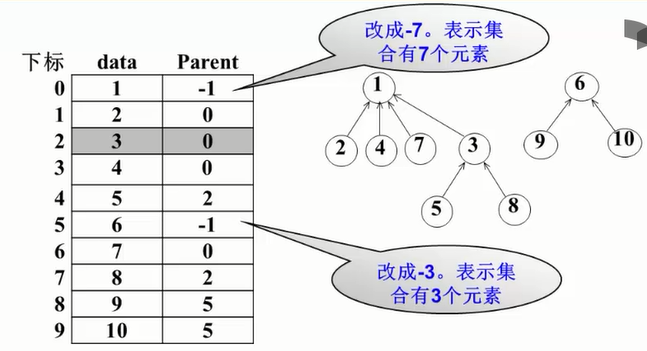#define MAXN 1000                  /* 集合最大元素个数 */
typedef int ElementType;           /* 默认元素可以用非负整数表示 */
typedef int SetName;               /* 默认用根结点的下标作为集合名称 */
typedef ElementType SetType[MAXN]; /* 假设集合元素下标从0开始 */

void Union( SetType S, SetName Root1, SetName Root2 )
{ /* 这里默认Root1和Root2是不同集合的根结点 */
/* 保证小集合并入大集合 */
if ( S[Root2] < S[Root1] ) { /* 如果集合2比较大 */
S[Root2] += S[Root1];     /* 集合1并入集合2  */
S[Root1] = Root2;
}
else {                         /* 如果集合1比较大 */
S[Root1] += S[Root2];     /* 集合2并入集合1  */
S[Root2] = Root1;
}
}

SetName Find( SetType S, ElementType X )
{ /* 默认集合元素全部初始化为-1 */
if ( S[X] < 0 ) /* 找到集合的根 */
return X;
else
return S[X] = Find( S, S[X] ); /* 路径压缩 */
}



展开全文• 数据结构C语言版链表的集合运算，包含交集、并集、差集。
• 集合set去重的,可变的,可迭代的,无序的 元素集合set的元素必须是可hash的约定set翻译为集合collection 翻译为集合类型,是一个大概念set定义 初始化set() -> new empty set objectset(iterable) -> new set ...
• 集合运算：交、并、补、差，判定一个元素是否属于某一集合 并查集：集合并、查某元素属于什么集合 并查集问题中集合存储如何实现？ 链式存储：树结构表示集合，树的每个结点代表一个集合元素 顺序存储：用数组...
• 该程序的功能是要能够实现集合的交、并、差的运算。并能够正确显示。 2、 本程序中集合的元素必须是数字类型的，可以是整数，不能够是字符型浮点型或者字符串等。 3、 由于一个集合中不应该出现相同的两个数，所以...
• 7. 集合运算 (单循环链表) 1.问题描述： 设有两个带头结点的单循环链表存储的集合A、B，其元素类型为字符或者整形，且以非递减方式存储，其头结点分别为ha、hb。要求下面各问题中的结果集合同样以非递减方式存储，...
• 提供数据结构集合的所有运算（c++类实现） 直接提供源代码，c++编译器可直接运行，楼主编辑采用Clion。 改代码设计数据结构集合的所有基本运算，包括交集，并集，差集，集合包含判断（子集判断）在集合中查找...c++ 链表 算法
• 数据结构C语言版顺序表的集合运算，顺序表交集 顺序表并集 顺序表差集。
• #include&lt;iostream&...//*判断相等运算*//void Pay(int,int);//*交运算*//void And(int,int);//*并运算*//int Fill(int,int);//*差运算*//int main(){int array1;void menu();cout&l...
• 实验三 基于线性表的集合运算 1、实验目的： （1）掌握线性表的使用方法。 （2）掌握线性表的顺序存储结构。 2、实验环境与设备： 已安装Visual Studio 2010（或其以上版本）集成开发环境的计算机。 3、实验原理： ...
• 三、数据结构分析 •••••••••••••••••••••••••••••••••••••••••••••••• 2 3.1、实现集合 ••••••••••••••••••••••••••••••••••...
• 1、集合的并、交和差运算 任务：编制一个能演示执行集合的并、交和差运算的程序。 要求: (1) 集合的元素限定为小写字母字符 [‘a’..’z’] 。 (2) 演示程序以用户和计算机的对话方式执行。 实现提示:以链表表示...
• 通过该实验，让学生复习巩固C语言中的循环结构、循环控制条件、分支结构和数组/链表、函数的调用等有关内容，体会到用数组存储集合时，需要记录集合元素的个数，否则输出结果会出现数据越界现象。 （2）实验内容 ...链表
• 提出一种新的数据立方体结构，通过索引和集合的交并运算来获得查询结果，特别是在进行区域查询时，避免了将区域分解为点后再依次进行点查询的方式，从而在保持较少的磁盘空间和较好的点查询响应速度的情况下，改善...
• 集合的表示 采用数组存储形式 typedef struct{ ...集合运算 1、查找某元素所在的集合 int Find(SetType S[],ElementType X){ int i; for(i=0;i<MaxSize && S[i].Data != X; i++); i......

# 数据结构集合运算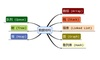数据结构 订阅